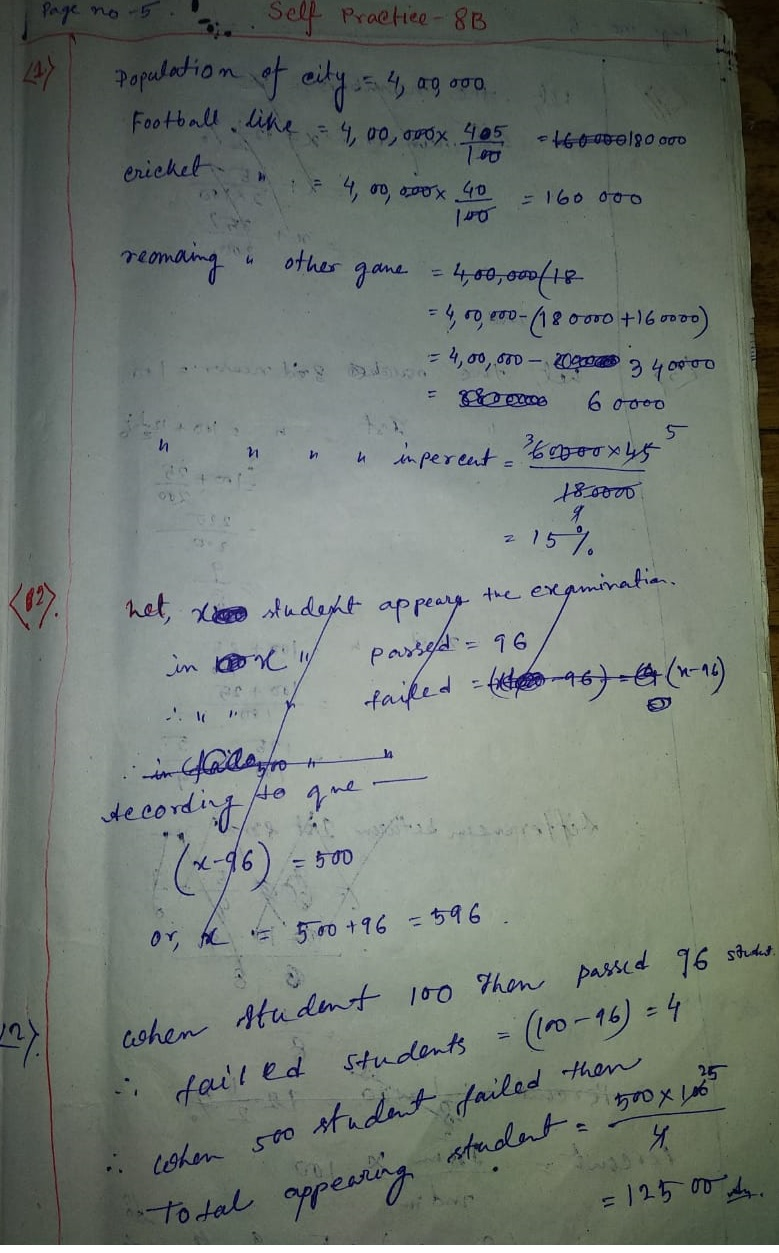# New Learning Composite Mathematics Class 8 SK Gupta Anubhuti Gangal Percentage and Its Application Chapter 8B Solution

## New Learning Composite Mathematics SK Gupta Anubhuti Gangal Class 8 Percentage and Its Application Self Practice 8B Solution

(1) If 45% people in a city like football, 40% like cricket and the remaining like other games, then what per cent of the people like other games? If the city has a population of 4,00,000 people, then find the number of people who like one of these games.

(2) In an examination, 96% of the students passed and 500 failed. How many students appeared at the examination?

Solution:(3) Two numbers are respectively 12 1 by 2% and 25% more than a third number. Find what percent is first number of the second number?

Solution:(4) A person invests Rs. 89856 in mutual funds, which is 26% of his annual income. What is the monthly income.

(5) The difference between 54% of a number and 26% of the same number is 22526. What is 66% of that number.

(6) When 125 is subtracted from a number, it reduces to its 37.5 per cent. What is 25 per cent of this number.

Solution:(7) A person spends 75% of his income, if his income increases by 20% and expenses increase by 15% then find the per cent increase in his savings.

(8) A shopkeeper first increased the price of an article by 25% and then by 20%. What is the total percent increase.

Solution:(9) A sum of Rs. 2236 is divided among A, B and C in such a way that A receives 25% more than C and C receives 25% less than B. What us A’s sharer in the amount?

(10) In a company, there are 75% skilled workers and the remaining are unskilled. 80% of skilled workers and 20% of unskilled workers are permanent. If the number of temporary workers is 126, then what is the number of workers?

Solution:(11) Ravi scored 30% marks and failed by 15 marks. Deepak scored 40% marks and obtained 35 marks more than those required to pass. Find the minimum per cent pass marks.

(12) Two candidates fought an election. One of them got 62% of the total votes and won by 432 votes. What is the total number of votes polled?

Solution:(13) In an election between two persons, 68 votes were declared invalid. The winning persons for 52% AND WON 98 VOTES. fIND THE TOTAL NUMBER OF VOTES POLLED.

Solution:Updated: March 20, 2020 — 1:20 pm